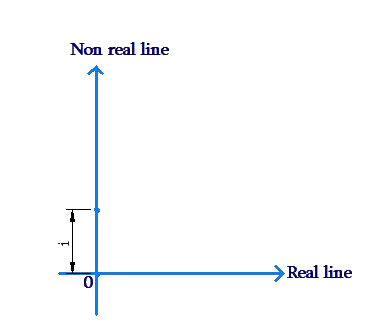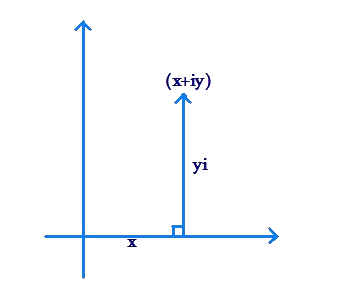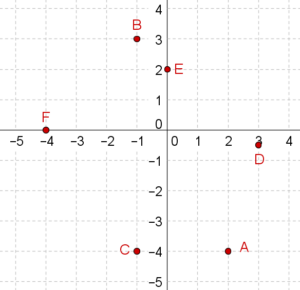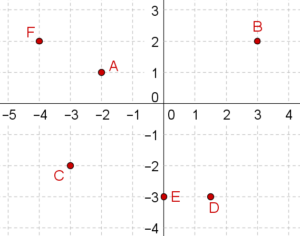# What is i ?

What is i ?

What exactly is the term i in the complex number $$x + iy$$ corresponding to the point $$P\left( {x,y} \right)$$? Clearly, i cannot be a real number, because if i was real, then $$x + iy$$ would also be real, and would lie on the Real Line itself. Thus, if i was real, then $$x + iy$$ would only represent points on the Real Line, rather than points in a plane.

If we want to represent points in a plane using numbers, i must be a non-real number, in the sense that it must not lie in the Real set. We interpret i as follows: i is one unit in the direction perpendicular to the Real Line:Thus, in the complex $$x + iy$$, the term iy becomes a non-real number: it represents y times i, that is, y units in the i direction. Hence $$x + iy$$ represents a point which can be reached by moving x units in the Real direction and y units in the i direction (from the origin):It also turns out that arithmetically, $${i^2} = - 1$$. In other words, i is the square-root of $$- 1$$. For now, don’t ask how! As we said earlier, the various different parts of the puzzle that is Complex Numbers will fall into place as you delve deeper into this subject.

At this point, just keep in mind that:

• i is a non-real number (it lies outside the Real set).

• i represents one unit perpendicular to the Real direction.

• yi represents y units perpendicular to the Real direction

• $$x + iy$$ represents the point $$\left( {x,y} \right)$$

• i is the square-root of $$- 1$$, or, $${i^2} = - 1$$

When we say that i is a non-real number, we do not mean that i does not exist or is a figment of our imagination. We mean to say that i is non-real in the sense that it does not like in the Real set. However, it is a perfectly valid mathematical entity. i is also known by the name iota.

Example 1: Represent each of the following points using complex numbers:

\begin{align}&A\left( {3,2} \right), \qquad\;\;\;\;\;\;\;\;\;\;\;\;B\left( { - 2,3} \right), \qquad\;\;\;\;\;\; C\left( {0,4} \right)\\&D\left( { - \frac{1}{2}, - \frac{3}{2}} \right), \qquad E\left( {\sqrt 2 , - \sqrt 2 } \right), \qquad F\left( {\pi ,0} \right) & \end{align}

Solution: A point with coordinates $$\left( {x,y} \right)$$ corresponds to the complex number $$x + yi$$. We have:

\begin{align}&A \equiv 3 + 2i, \qquad \;\;\;\;\;\;\;B \equiv - 2 + 3i, \qquad\;\;\; C \equiv 4i\\&D \equiv - \frac{1}{2} - \frac{3}{2}i,\qquad E \equiv \sqrt 2 - \sqrt 2 i,\qquad F \equiv \pi \end{align}

Note that C corresponds to a purely non-real number (there is no real part), while F corresponds to a purely real number (there is no non-real part). A purely non-real number is also known as a purely imaginary number.

Example 2: Specify the points (in coordinate form) corresponding to the following complex numbers:

$\begin{array}{l}A\left( {4 - 3i} \right), & B\left( { - 7 - 2i} \right), & C\left( {2i - 1} \right)\\D\left( {i + \sqrt 2 } \right), & E\left( { - \frac{i}{{\sqrt 2 }} - \sqrt 3 } \right)\\F\left( {\pi i} \right), & G\left( {\frac{1}{{\sqrt 3 }}} \right)\end{array}$

Solution: A complex number $$x + yi$$ corresponds to the point $$\left( {x,y} \right)$$ in the plane. Thus,

$\begin{array}{l}A \equiv \left( {4, - 3} \right), & B \equiv \left( { - 7, - 2} \right), & C \equiv \left( { - 1,2} \right)\\D \equiv \left( {\sqrt 2 ,1} \right), & E \equiv \left( { - \sqrt 3 , - \frac{1}{{\sqrt 2 }}} \right)\\F \equiv \left( {0,\pi } \right), & G \equiv \left( {\frac{1}{{\sqrt 3 }},0} \right)\end{array}$

Note, for example, the coordinate form of C. The number was given as $$C\left( {2i - 1} \right)$$. In the standard complex form, this number can be written as $$C\left( { - 1 + 2i} \right)$$.

Example 3: Plot the following complex numbers in the complex plane:

$\begin{array}{l}A\left( {2 - 4i} \right), & B\left( {3i - 1} \right), & C\left( { - 1 - 4i} \right)\\D\left( { - \frac{i}{2} + 3} \right), & E\left( {2i} \right), & F\left( { - 4} \right)\end{array}$

Solution: The six points are plotted below:Example 4: Write the complex numbers corresponding to each of the following points:Solution: Any point $$\left( {x,y} \right)$$ corresponds to the complex number $$x + yi$$:

$\begin{array}{l}A \equiv \left( { - 2,1} \right) \equiv - 2 + i & \\B \equiv \left( {3,2} \right) \equiv 3 + 2i & \\C \equiv \left( { - 3, - 2} \right) \equiv - 3 - 2i\\D \equiv \left( {1.5, - 3} \right) \equiv 1.5 - 3i\\E \equiv \left( {0, - 3} \right) \equiv - 3i\\F \equiv \left( { - 4,2} \right) \equiv - 4 + 2i\end{array}$

We note the following:

1. Any real number x can be written as $$x + i0$$. In other words, a real number can also be thought of as a complex number in which the non-real part is 0. Thus, every real number is a complex number. Put differently, the Real set is a subset of the Complex set. This should be geometrically obvious as well: the Real Line is a subset of the Complex Plane.

2. An arbitrary complex number is generally represented using the letter z. If $$z = x + yi$$, then:

a) x is known as the real part of z, and is denoted by Re(z)

b) y is known as the imaginary part of z, and is denoted by Im(z)

For example, if $$z = - 2 - 7i$$, then $${\mathop{\rm Re}\nolimits} \left( z \right) = - 2$$ and $${\mathop{\rm Im}\nolimits} \left( z \right) = - 7$$. Basically, the real and imaginary parts of a complex number are the same as the coordinates of the corresponding point.

Complex Numbers
grade 10 | Questions Set 1
Complex Numbers
Complex Numbers
Complex Numbers
grade 10 | Questions Set 2
More Important Topics
Numbers
Algebra
Geometry
Measurement
Money
Data
Trigonometry
Calculus
More Important Topics
Numbers
Algebra
Geometry
Measurement
Money
Data
Trigonometry
Calculus
Learn from the best math teachers and top your exams

• Live one on one classroom and doubt clearing
• Practice worksheets in and after class for conceptual clarity
• Personalized curriculum to keep up with school